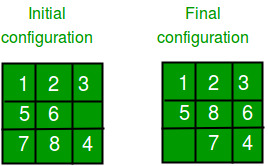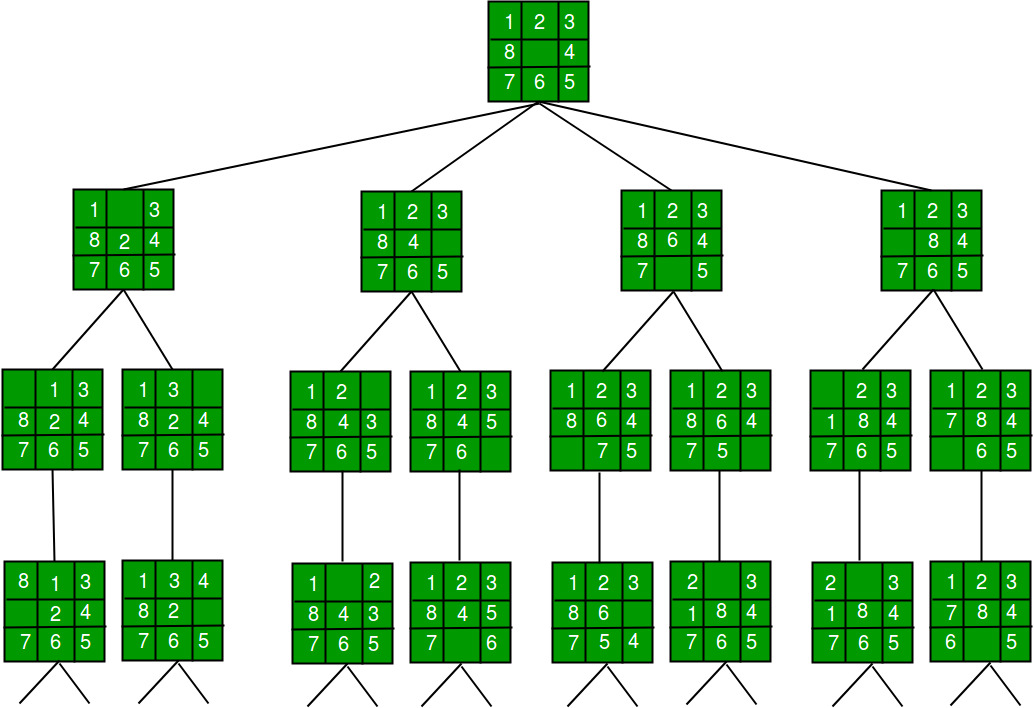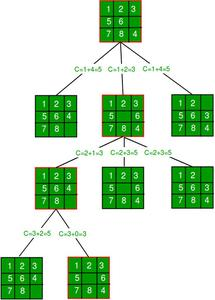Related Articles
8 puzzle Problem using Branch And Bound
• Difficulty Level : Hard
• Last Updated : 24 Mar, 2021

We have introduced Branch and Bound and discussed the 0/1 Knapsack problem in the below posts.

In this puzzle solution of the 8 puzzle problem is discussed.
Given a 3×3 board with 8 tiles (every tile has one number from 1 to 8) and one empty space. The objective is to place the numbers on tiles to match the final configuration using the empty space. We can slide four adjacent (left, right, above, and below) tiles into the empty space.
For example,1. DFS (Brute-Force)
We can perform a depth-first search on state-space (Set of all configurations of a given problem i.e. all states that can be reached from the initial state) tree.State Space Tree for 8 Puzzle

In this solution, successive moves can take us away from the goal rather than bringing us closer. The search of state-space tree follows the leftmost path from the root regardless of the initial state. An answer node may never be found in this approach.
2. BFS (Brute-Force)
We can perform a Breadth-first search on the state space tree. This always finds a goal state nearest to the root. But no matter what the initial state is, the algorithm attempts the same sequence of moves like DFS.
3. Branch and Bound
The search for an answer node can often be speeded by using an “intelligent” ranking function, also called an approximate cost function to avoid searching in sub-trees that do not contain an answer node. It is similar to the backtracking technique but uses a BFS-like search.
There are basically three types of nodes involved in Branch and Bound
1. Live node is a node that has been generated but whose children have not yet been generated.
2. E-node is a live node whose children are currently being explored. In other words, an E-node is a node currently being expanded.
3. Dead node is a generated node that is not to be expanded or explored any further. All children of a dead node have already been expanded.
Cost function:
Each node X in the search tree is associated with a cost. The cost function is useful for determining the next E-node. The next E-node is the one with the least cost. The cost function is defined as

```   C(X) = g(X) + h(X) where
g(X) = cost of reaching the current node
from the root
h(X) = cost of reaching an answer node from X.```

The ideal Cost function for an 8-puzzle Algorithm :
We assume that moving one tile in any direction will have a 1 unit cost. Keeping that in mind, we define a cost function for the 8-puzzle algorithm as below:

```   c(x) = f(x) + h(x) where
f(x) is the length of the path from root to x
(the number of moves so far) and
h(x) is the number of non-blank tiles not in
their goal position (the number of mis-
-placed tiles). There are at least h(x)
moves to transform state x to a goal state```

An algorithm is available for getting an approximation of h(x) which is an unknown value.
Complete Algorithm:

```/* Algorithm LCSearch uses c(x) to find an answer node
* LCSearch uses Least() and Add() to maintain the list
of live nodes
* Least() finds a live node with least c(x), deletes
it from the list and returns it
* Add(x) adds x to the list of live nodes
* Implement list of live nodes as a min-heap */

struct list_node
{
list_node *next;

// Helps in tracing path when answer is found
list_node *parent;
float cost;
}

algorithm LCSearch(list_node *t)
{
// Search t for an answer node
// Input: Root node of tree t
// Output: Path from answer node to root
if (*t is an answer node)
{
print(*t);
return;
}

E = t; // E-node

Initialize the list of live nodes to be empty;
while (true)
{
for each child x of E
{
if x is an answer node
{
print the path from x to t;
return;
}
Add (x); // Add x to list of live nodes;
x->parent = E; // Pointer for path to root
}

if there are no more live nodes
{
print ("No answer node");
return;
}

// Find a live node with least estimated cost
E = Least();

// The found node is deleted from the list of
// live nodes
}
}```

The below diagram shows the path followed by the above algorithm to reach the final configuration from the given initial configuration of the 8-Puzzle. Note that only nodes having the least value of cost function are expanded.## C++14

 `// Program to print path from root node to destination node``// for N*N -1 puzzle algorithm using Branch and Bound``// The solution assumes that instance of puzzle is solvable``#include ``using` `namespace` `std;``#define N 3` `// state space tree nodes``struct` `Node``{``    ``// stores the parent node of the current node``    ``// helps in tracing path when the answer is found``    ``Node* parent;` `    ``// stores matrix``    ``int` `mat[N][N];` `    ``// stores blank tile coordinates``    ``int` `x, y;` `    ``// stores the number of misplaced tiles``    ``int` `cost;` `    ``// stores the number of moves so far``    ``int` `level;``};` `// Function to print N x N matrix``int` `printMatrix(``int` `mat[N][N])``{``    ``for` `(``int` `i = 0; i < N; i++)``    ``{``        ``for` `(``int` `j = 0; j < N; j++)``            ``printf``(``"%d "``, mat[i][j]);``        ``printf``(``"\n"``);``    ``}``}` `// Function to allocate a new node``Node* newNode(``int` `mat[N][N], ``int` `x, ``int` `y, ``int` `newX,``              ``int` `newY, ``int` `level, Node* parent)``{``    ``Node* node = ``new` `Node;` `    ``// set pointer for path to root``    ``node->parent = parent;` `    ``// copy data from parent node to current node``    ``memcpy``(node->mat, mat, ``sizeof` `node->mat);` `    ``// move tile by 1 position``    ``swap(node->mat[x][y], node->mat[newX][newY]);` `    ``// set number of misplaced tiles``    ``node->cost = INT_MAX;` `    ``// set number of moves so far``    ``node->level = level;` `    ``// update new blank tile cordinates``    ``node->x = newX;``    ``node->y = newY;` `    ``return` `node;``}` `// bottom, left, top, right``int` `row[] = { 1, 0, -1, 0 };``int` `col[] = { 0, -1, 0, 1 };` `// Function to calculate the number of misplaced tiles``// ie. number of non-blank tiles not in their goal position``int` `calculateCost(``int` `initial[N][N], ``int` `final[N][N])``{``    ``int` `count = 0;``    ``for` `(``int` `i = 0; i < N; i++)``      ``for` `(``int` `j = 0; j < N; j++)``        ``if` `(initial[i][j] && initial[i][j] != final[i][j])``           ``count++;``    ``return` `count;``}` `// Function to check if (x, y) is a valid matrix cordinate``int` `isSafe(``int` `x, ``int` `y)``{``    ``return` `(x >= 0 && x < N && y >= 0 && y < N);``}` `// print path from root node to destination node``void` `printPath(Node* root)``{``    ``if` `(root == NULL)``        ``return``;``    ``printPath(root->parent);``    ``printMatrix(root->mat);` `    ``printf``(``"\n"``);``}` `// Comparison object to be used to order the heap``struct` `comp``{``    ``bool` `operator()(``const` `Node* lhs, ``const` `Node* rhs) ``const``    ``{``        ``return` `(lhs->cost + lhs->level) > (rhs->cost + rhs->level);``    ``}``};` `// Function to solve N*N - 1 puzzle algorithm using``// Branch and Bound. x and y are blank tile coordinates``// in initial state``void` `solve(``int` `initial[N][N], ``int` `x, ``int` `y,``           ``int` `final[N][N])``{``    ``// Create a priority queue to store live nodes of``    ``// search tree;``    ``priority_queue, comp> pq;` `    ``// create a root node and calculate its cost``    ``Node* root = newNode(initial, x, y, x, y, 0, NULL);``    ``root->cost = calculateCost(initial, final);` `    ``// Add root to list of live nodes;``    ``pq.push(root);` `    ``// Finds a live node with least cost,``    ``// add its childrens to list of live nodes and``    ``// finally deletes it from the list.``    ``while` `(!pq.empty())``    ``{``        ``// Find a live node with least estimated cost``        ``Node* min = pq.top();` `        ``// The found node is deleted from the list of``        ``// live nodes``        ``pq.pop();` `        ``// if min is an answer node``        ``if` `(min->cost == 0)``        ``{``            ``// print the path from root to destination;``            ``printPath(min);``            ``return``;``        ``}` `        ``// do for each child of min``        ``// max 4 children for a node``        ``for` `(``int` `i = 0; i < 4; i++)``        ``{``            ``if` `(isSafe(min->x + row[i], min->y + col[i]))``            ``{``                ``// create a child node and calculate``                ``// its cost``                ``Node* child = newNode(min->mat, min->x,``                              ``min->y, min->x + row[i],``                              ``min->y + col[i],``                              ``min->level + 1, min);``                ``child->cost = calculateCost(child->mat, final);` `                ``// Add child to list of live nodes``                ``pq.push(child);``            ``}``        ``}``    ``}``}` `// Driver code``int` `main()``{``    ``// Initial configuration``    ``// Value 0 is used for empty space``    ``int` `initial[N][N] =``    ``{``        ``{1, 2, 3},``        ``{5, 6, 0},``        ``{7, 8, 4}``    ``};` `    ``// Solvable Final configuration``    ``// Value 0 is used for empty space``    ``int` `final[N][N] =``    ``{``        ``{1, 2, 3},``        ``{5, 8, 6},``        ``{0, 7, 4}``    ``};` `    ``// Blank tile coordinates in initial``    ``// configuration``    ``int` `x = 1, y = 2;` `    ``solve(initial, x, y, final);` `    ``return` `0;``}`

Output :

```1 2 3
5 6 0
7 8 4

1 2 3
5 0 6
7 8 4

1 2 3
5 8 6
7 0 4

1 2 3
5 8 6
0 7 4```

Sources:
www.cs.umsl.edu/~sanjiv/classes/cs5130/lectures/bb.pdf
https://www.seas.gwu.edu/~bell/csci212/Branch_and_Bound.pdf
This article is contributed by Aditya Goel. If you like GeeksforGeeks and would like to contribute, you can also write an article and mail your article to contribute@geeksforgeeks.org. See your article appearing on the GeeksforGeeks main page and help other Geeks.
Please write comments if you find anything incorrect, or you want to share more information about the topic discussed above

Attention reader! Don’t stop learning now. Get hold of all the important DSA concepts with the DSA Self Paced Course at a student-friendly price and become industry ready.

My Personal Notes arrow_drop_up